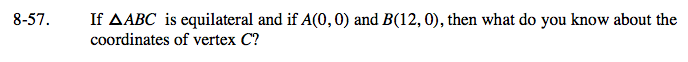Home > GC > Chapter 8 > Lesson 8.1.5 > Problem8-57

8-57.

If ΔABC is equilateral and if A(0, 0) and B(12, 0), then what do you know about the coordinates of vertex C? 8-57 HW eTool (Desmos). Homework Help ✎Use the eTool below to create your equilateral triangles.
Click the link at right for the full version of the eTool: GC 8-57 HW eTool

Notice the position of the third vertex for both triangles. They both have an x-coordinate of 6.

Use the patterns you learned for special right triangles to find the height of the congruent equilateral triangles.

$\text{Vertex }C\text{ can be at }(6,6\sqrt{3})\text{ or }(6,-6\sqrt{3}).$